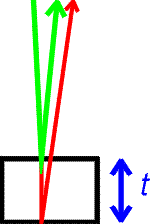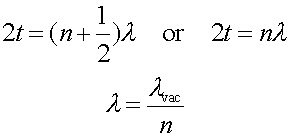Thin films

Consider monochromatic light which falls from above on a thin film of material. Some of the reflected light comes off the upper surface and some reflects off the lower surface as shown to the right. An interference maximum results when the path difference, twice the thickness 2t, is an integral (or half integral depending on conditions discussed below) number of wavelengths.One must remember that the relavant wavelength is that in the material of the film, which is related to the wavelength in vacuum by the inverse of the index of refraction n.

Light will either reflect with a 180 degree phase change if it reflects off a surface with higher index of refraction. For example, if the film is water surrounded on both sides by air, the upper reflection gets a 180 degree surface, but not the lower one, and one uses the formula with the extra 1/2 above. If there is vacuum above, liquid in the film, and glass below of an even higher index, one uses the formula without the 1/2.

Examples     Interference and diffraction's index